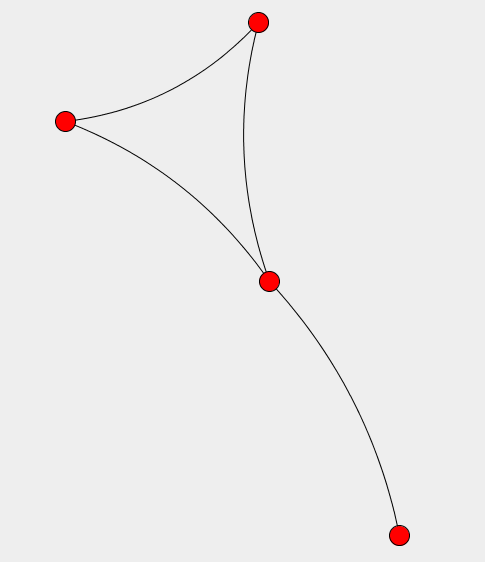# DMelt:Math/7 Graphs

Limitted access. First login to DataMelt if you are a full DataMelt member. Then login to HandWiki as a user.

## Graphs

Graphs belong to a branch of mathematics, graph theory. For data analysis that requires searches of particular patterns, graph-based data mining becomes an important technique. Read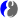Graph theory.

In real life, most of the data we have to deal with can be represented as graphs. A typical graph consists of vertices (nodes, cells), and of edges that are the connecting lines between the nodes. Analysis of graphs includes determining certain details about the graph structure. For example, determining all routes or the shortest path between two nodes or cells.

DMelt supports the following graph libraries

These libraries support graph creation using cells and edges, as well as their visualization. Note that DMelt includes a Swing GUI to draw graphs. Look at the menu [Tools]->[Graph Editor]. In this section we will show how to draw Graphs and analyze them.

## Drawing graphs

The drawing of graphs in DMelt is based on the Java classjhplot.HGraph. Here is a simple example to draw vertices and edges: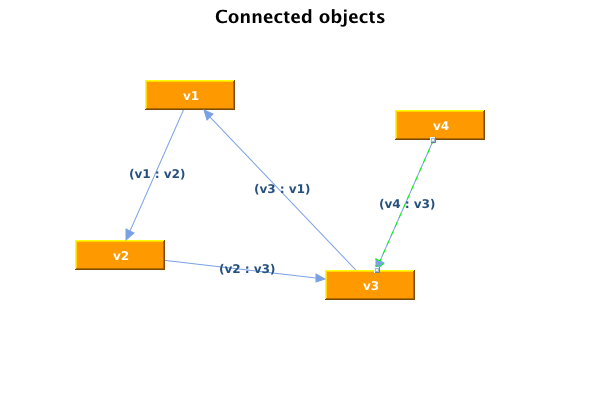The code is shown below:

The above example shows how to build a graph using a predefined methods ofjhplot.HGraph. Generally, there are two types of programs that can be used to work with graphs:

Let us consider the latter case:

In this example we create a graph, and work with them, and later visualize. It creates the following image: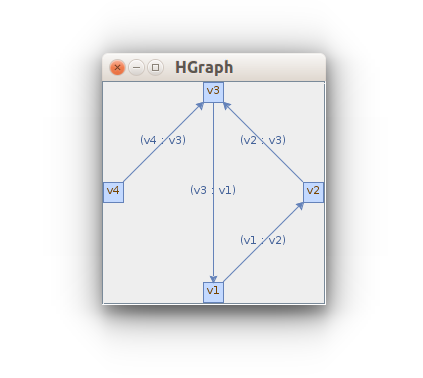## Analyzing the graphs

There are a number of algorithms that can be used to work with the graphs. Let us consider a typical example: finding a shortest path between two verticies, Look at the API summaryorg.jgrapht.alg.shortestpath.package-summary andorg.jgrapht.alg.package-summary.

We will use the graphical approach when we construct the graph on the canvas, access it, and run a few algorithms to find the shortest path:

This example prints the pass usingorg.jgrapht.alg.shortestpath.DijkstraShortestPath (Dijkstra Shortest Path) algorithm.

## Weighted graphs

In many applications, each edge of a graph can have an associated numerical value, called a weight Weighted graphs may be either directed or undirected. The weight of an edge is often referred to as the "cost" of the edge. For example, the weight may be a measure of the length of a route, the used energy that is required to drive between locations along a route, etc.

Here is an example of how to build a graph with weighted edges. Then we run several algorithms to identify a path with smallest weight, For example, KShortestPaths algorithm finds a path that has smallest weight (event although it has several edges). We also run the BellmanFordShortestPath algorithm that can be used with graph with edges.

The last line of the code prints all paths, ordered them according to the weight.

## Graph input and output

Graphs can be saved and can be read back from a files. For example, graphs can be saved to GraphML (i.e. XML-like) format using as shown in this example:

Here we create a weighted graph and save the into GraphXL.

One convenient method is to save files is to use CVS files. You do not need any particular library to do this. Here we show how to save and restore a weighted graph in a CVS using the Python syntax:

The result of this script is a graph which is is identical to the original one.

## Using Medusa

Let us give another complex example: building a graph using the classjhplot.HMedusa, that calls the Medusa Java library. This Java library is a powerful framework for visualization of interactive graphs and, primary, for clustering analysis of large-scale biological networks. Here is is an image of simple interactive graph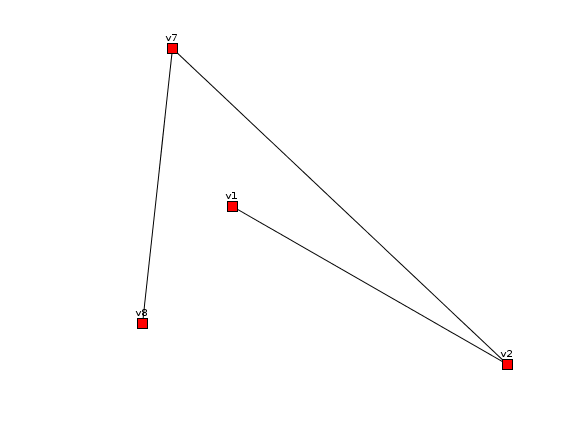with the code shown bellow:

One can also build a complex graphs from files as will shown in the Dmelt code example. Read DMelt:ExternalGuides/Medusa.

## Using Jung

Jung library (see Jung API) is another powerful library to build and visualize graphs. To work with it, you will need a to 1) Build a graph; 2) Create a visualizer 3) Attach a visualizer to a Java JFrame. This small code shows this:

with the image given bellow: